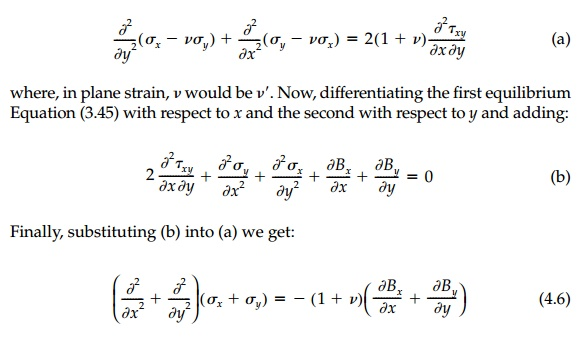Home | | Mechanics of Solids | Two Dimensional Stress Formulation

# Two Dimensional Stress Formulation

In 2D it is easy to follow the steps only outlined in 3D for Equations (4.4) and (4.5) for the direct determination of stress formulation.

Two-Dimensional Stress Formulation

In 2D it is easy to follow the steps only outlined in 3D for Equations (4.4) and (4.5) for the direct determination of stress formulation. Substituting the stress-strain relationships [Equation (2.4)] into the strain compatibility [Equation (2.7)] gives:Equation (4.6) for nonconstant body forces is known as the 'compatibility equation in terms of stress,' but it actually combines compatibility, equilib-rium, and stress-strain relations.

This development is remarkable. For a linear stress-strain material and constant body forces, the 2D field equationsare independent of material properties!

Thus:

a.  In-plane stresses are identical for any given geometry, loading, and BCs (i.e., stresses in similar steel, concrete, wood structures are the same).

b.  This, in turn, allows the experimental determination of stress fields using plastic or other materials.

c.  The in-plane stresses are the same for plane strain or plane stress (which again is very nice for experimental work since plane strain is difficult to achieve in the laboratory).

d.  Linear viscoelastic stress fields are the same for constant load (creep) and do not change with time.

Two-dimensional solutions are, therefore, very general and powerful.* Conceptually it is usually easier to think in terms of these stress equations rather than their displacement counterparts and we will concentrate on this approach.

It is also remarkable that the mathematics and our previous discussion of the physical importance of the invariants (independent of coordinate system) seem to be converging. All of a sudden, the first invariant (the mean stress as the center of Mohr's Circle) has appeared and in the form of the most com-mon, most studied, best understood differential equation in the universe:** the Laplace or harmonic equation. The isotropic component seems to have an equation of its own, uncoupled from the deviatoric component, which must be described by the equilibrium Equations (2.45).

Study Material, Lecturing Notes, Assignment, Reference, Wiki description explanation, brief detail
Civil : Principles of Solid Mechanics : Strategies for Elastic Analysis and Design : Two Dimensional Stress Formulation |

Related Topics